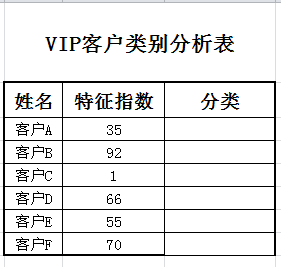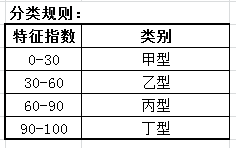• Excel VBA 函数返回值
万次阅读
2020-02-11 13:29:52

# Excel VBA 函数返回值

## Sub 定义一个过程

VB的函数定义格式与C有很大区别：
格式：

Sub 过程名(参数列表 ... )
过程体
End Sub


## Function 定义一个函数

Function 函数名(参数列表 ... )
函数体
函数名 = 返回值
End Function


实例代码

Sub Main()
temp = Application.InputBox(Prompt:= _
"Please enter the temperature in degrees F.", Type:=1)
MsgBox "The temperature is " & Celsius(temp) & " degrees C."
End Sub

Function Celsius(fDegrees)
Celsius = (fDegrees - 32) * 5 / 9
End Function


## 调用Sub过程或者Function函数

调用函数时，有两种形式：

1. 可以直接将参数放在函数名后，英文逗号 ‘,’ 作为参数分隔符，不需要加圆括号。
此种调用方式，将舍弃函数返回值。
Sub Main()
MultiBeep 56
Message
End Sub

Sub MultiBeep(numbeeps)
For counter = 1 To numbeeps
Beep
Next counter
End Sub

Sub Message()
MsgBox "Time to take a break!"
End Sub

1. 使用类c语言的方式，参数后加圆括号，用以包含参数。
Sub Main()
HouseCalc 99800, 43100
Call HouseCalc(380950, 49500)
End Sub

Sub HouseCalc(price As Single, wage As Single)
If 2.5 * wage <= 0.8 * price Then
MsgBox "You cannot afford this house."
Else
MsgBox "This house is affordable."
End If
End Sub

1. 函数名前，可加Call关键字，亦可省略。

References:

Microsoft Docs | Calling Sub and Function proceduresexcel
更多相关内容
• EXCEL VBA函数手册 很实用的EXCEL VBA函数手册值得收藏
• vba 函数速查手册，做成vb窗口形式 点击按钮就可以查看
• Abs 函数 ...Sgn 函数、数学函数中的Abs 函数、Atn 函数、Cos 函数、Exp 函数、Fix 函数、Int 函数、Log 函数、Rnd 函数、Sgn 函数、Sin 函数、Sqr 函数、Tan 函数、导出的数学函数： 导出的数学函数
• VBA函数大全(自己整理的) 里面整理了vba所有内置函数 方便查阅
• 实用文档 VB 实战 excel 学习 excel 高级班 excel 表格的基本操作 excel 表格的各函数的基本操作 Excel 常用 VBA 函数实用经典案例 ASC 函数 一题目 要求编写一段代码运行后得到字符串 Excel的首字母和 e的 ASCII值 ...
• ## VBA函数定义及说明

千次阅读 2020-06-24 11:18:28
函数定义，函数返回对象，默认参数，不定长参数

# 函数定义

VBA定义的函数可以在工作表使用，如果是在加载插件中定义函数，本机所有打开工作簿都可以使用该函数，当然可以在过程sub中调用函数；

VBA函数与sub过程不同的是，函数有返回内容；过程和函数都可以传入参数。
函数使用Function关键字定义，定义规则如下：
Function 函数名称(形参及类型)
函数主体
函数名称= 函数返回
End Function
示例：

'定义一个数值平方的函数，形参：a，形参a类型：long，函数返回：a ^ 2；函数名称：test
Function test(a as long)
test = a ^ 2
End Function

'定义全局函数,使用public关键字，这个关键字跟变量定义是一致的。后面跟的as long是返回类型
Public Function test(a as long) as long
test = a ^ 2
End Function


# 传值和传引用

函数或方法传值使用关键字ByVal，传引用使用关键字ByRef

Sub num_print()
Dim i, num As Long  ' 定义一个变量
num = 0
For i = 1 To 10
Debug.Print num     ' 函数参数是传引用，会依次打印1,2,3,,,,10
Next
End Sub

a = a + 1
End Function


如果上述函数参数为传值ByVal，则函数不影响方法num_print中变量num的改变，全打印0；

# 函数返回对象

函数也可以返回对象，返回对象要使用set关键字；
示例：返回字典

Function aa()
Dim d As Object

Set d = CreateObject("scripting.dictionary")
today = Date
the_month_date = CDate(Year(Date) & "-" & Month(Date) & "-" & 20)   '这个月的20号
last_month_date = Application.WorksheetFunction.EDate(the_month_date, -1)  '上个月的20号
d("today") = today
d("the_month_date") = the_month_date
d("last_month_date") = last_month_date
d("the_month") = Month(last_month_date)      '这个月
d("last_month") =Month(Date)  '上个月

Set aa = d    	'返回对象使用set关键字
End Function

'函数调用
sub test1()
dim d1 as object

set d1 = aa()
debug.print d1("today")    '打印字典键today对应的值
end sub


# 使用默认参数

函数传入参数格式：形参 as 参数类型 = 参数默认值
示例：正则提取函数

Function regexp(rg As Variant, str As String, Optional mat As Byte = 0, Optional group As Variant = Empty)
'Optional表示参数不是必需的关键字。如果使用了该选项，则参数表中该参数后的参数都必须是可选的，而且必须都使用 Optional 关键字声明。
Dim re As Object
Set re = CreateObject("vbscript.regexp")
With re
.Global = True
.Pattern = str
If re.test(rg) Then
If group = Empty Then
regexp = re.Execute(rg)(mat)
Else
regexp = re.Execute(rg)(mat).submatches(group)
End If
End If
End With
Set re = Nothing
End Function


# 使用不定长参数

形参及类型固定写法：ParamArray 参数名称() As Variant（必须放在参数最后面）
示例：只要有一个单元格为空，返回空字符串

Function if_blank(goal_rg As Variant, ParamArray rngs() As Variant)
Dim rg
For Each rg In rngs
If rg.Value = "" Then
if_blank = ""
Exit Function
End If
Next
if_blank = goal_rg
End Function


示例：单元格求和sum

Function rng_sum(ParamArray values() As Variant)
Dim result As Variant
Dim val0 As Variant    ' for循环里的变量必须是变体型变量，否则会报错
result = 0
For Each val0 In values
For Each val1 In val0
result = result + val1
Next
Next
rng_sum = result
End Function

'然后我们在工作表里写了这么一个函数
=rng_sum(K21:L21,M22:N22,L23:N23)


# 一些函数示例

## text_split：字符串分割

EXCEL里面没有split函数，可以使用vba定义该函数，在工作表内使用

Function text_split(str As String, sep As String, index As Long)
' 参数：str:被分割的字符串,sep:分隔符,index:分割后返回数组该索引的值，如果小于0返回数组
' 样例：text_split("abc,de,fg",",")(1)  返回：de

If index >= 0 Then
text_split = Split(str, sep)(index)
Else
text_split = Split(str, sep)
End If
End Function


## file_exists：判断文件是否存在

判断文件是否存在，dir函数可以使用通配符：*

Function file_exists(full_name As String) As Boolean
file_exists = (Dir(full_name) <> "")
End Function


## basename：路径提取文件名

传入一个带路径完整的文件名，返回文件名，比如：test.xlsx

Function basename(full_name)
' Application.PathSeparator：反斜杠
' basename("d:/filedir/text.xlsx")，返回：text.xlsx
Dim arr As Variant
arr = Split(full_name, Application.PathSeparator)
basename = arr(UBound(arr))
End Function


## sheet_exists：工作表是否存在

Function sheet_exists(sheet_name As Variant) As Boolean
' 传入工作表名称，返回是否存在：boolean
' sheet_exists("工作表2")
Dim st As Object
On Error Resume Next
Set st = ActiveWorkbook.Sheets(sheet_name)
If Err.Number = 0 Then   ' 如果没有报错，返回true
sheet_exists = True
Else
sheet_exists = False
End If


## workbook_is_open:工作表是否存在

Function workbook_is_open(wb_name As Variant) As Boolean
' 传入工作簿名称，返回是否打开：boolean
' sheet_exists("工作表2")
Dim st As Object
On Error Resume Next
Set st = Workbooks(wb_name)
If Err.Number = 0 Then   ' 如果没有报错，返回true
workbook_is_open = True
Else
workbook_is_open = False
End If


## text_join：split的反函数

该函数在Excel2019版已经引入，早期的版本可以通过自定义函数实现

Function text_join(sep As String, is_skip_blank As Boolean, ParamArray ranges() As Variant)
' sep：分隔符，is_skip_blank:是否跳过空值，ranges：数组
Dim rngs, sub_rng As Variant
Dim s As String
s = ""
For Each rngs In ranges
For Each sub_rng In rngs
If is_skip_blank = True Then   ' 是否跳过空格
If Len(sub_rng) > 0 Then
s = s & sep & Rng
End If
Else
s = s & sep & Rng
End If
Next
Next
text_join = Replace(s, sep, "", 1, 1)     ' 把开头的分隔符去掉
End Function


## ifs：多判断

该函数在excel2019版本后才有，早期的版本可以在vba中定制；无须重复if嵌套

Function udf_ifs(ParamArray args() As Variant)
Dim i As Byte
Dim args_len As Byte

args_len = UBound(args)   ' 参数索引下标从0开始
If args_len < 1 Then Exit Function

For i = 0 To UBound(args) Step 2
If args(i) = True Then
udf_ifs = args(i + 1)   ' 如果参数是true，返回后面一个参数值
Exit Function
End If
Next

' 如果都没有是，参数个数是基数，返回最后一个参数
If args_len Mod 2 = 0 Then udf_ifs = args(args_len): Exit Function

udf_ifs = "#N/A"      ' 参数是偶数，且没有true对象，返回错误值
End Function


## range_workbook_name：返回单元格所在的工作簿名称

返回单元格所在工作簿的名称，parent表示父对象，比如单元格的父对象是工作表，工作表的父对象是工作簿，这里调用了两次

Function range_workbook_name(rng As Variant) As String
range_workbook_name = rng.Parent.Parent.Name
End Function


## text_speak：说出文本

使用的是Excel的文本转化成语音的转化生成器，讲述传入的字符串

Function text_speak(text)
' Application.Speech.Speak ("hello alice")
Application.Speech.Speak (text)
text_speak = text
End Function


## is_like：模式匹配

使用vba的like函数，类似于sql中的like，like中pattern参数的字符

pattern字符解释
任意单个字符
*0个或多个字符
#任意单个数字（0-9）
[charlist]字符列表中的任意单个字符
[!charlist]不在字符列表中的任意单个字符
Function is_like(str As String, pattern As String) As Boolean
is_like = str Like pat
End Function

展开全文• 文章目录VBA的函数excel函数对应的vba函数countavlookupexcel特有函数和vba特有函数vba 字符串函数instrsplitvba 常用函数错误处理控制输入的格式自己写函数带参数的sub代码复用 没完成的任务：张三李四登录p=8 VBA...

函数

### 文章目录

没完成的任务：张三李四登录p=8

# VBA的函数

熟悉的函数可以用，有哪些新的函数

## excel函数对应的vba函数

### counta

函数计算范围中不为空的单元格的个数。

=counta(a1:a10)
' 指定某个工作表的某个区域
=counta(重庆!A:A)-1


vba中使用普通方法，计算不为空的单元格：

Sub tongji()
Dim k, i As Integer
alrow = Sheet2.Range("a65525").End(xlUp).Row
For i = 1 To alrow
If Sheet2.Cells(i, 1) <> "" Then
k = k + 1
End If

Next
Sheet1.Range("d26") = k - 1
End Sub


vba中调用函数快速解决：


Sheet1.Range("d26") = WorksheetFunction.CountA(Sheet2.Range("a:a"))-1


例子：

Sub tongji()
Dim k, kboy, kgirl As Integer
For i = 2 To Sheets.Count
k = k + WorksheetFunction.CountA(Sheets(i).Range("a:a"))
kboy = kboy + WorksheetFunction.CountIf(Sheets(i).Range("f:f"), "男")
kgirl = kgirl + WorksheetFunction.CountIf(Sheets(i).Range("f:f"), "女")
Next
Sheet1.Range("d26") = k
Sheet1.Range("d27") = kboy
Sheet1.Range("d28") = kgirl

End Sub


### vlookup

函数功能

vlookup(找什么，在哪一块找(块的第一列会和找什么一一比较)，找到以后返回第几列，精确匹配吗(0表示精确))，



使用函数

=VLOOKUP(D9,重庆!A:H,5,0)


使用vba代码的案例：
！！ 如果函数找不到，会返回#value，但是vba找不到，程序会奔溃！！！

Sub chaxun()
Dim k As Integer
On Error Resume Next
Sheet1.Range("d14,d16,d18,d20,d22").ClearContents
For i = 2 To Sheets.Count
Sheet1.Range("d14") = WorksheetFunction.VLookup(Sheet1.Range("d9"), Sheets(i).Range("a:H"), 5, 0)
Sheet1.Range("d16") = WorksheetFunction.VLookup(Sheet1.Range("d9"), Sheets(i).Range("a:H"), 6, 0)
Sheet1.Range("d18") = WorksheetFunction.VLookup(Sheet1.Range("d9"), Sheets(i).Range("a:H"), 3, 0)
Sheet1.Range("d20") = WorksheetFunction.VLookup(Sheet1.Range("d9"), Sheets(i).Range("a:H"), 8, 0)
If Sheet1.Range("d14") <> "" Then
Sheet1.Range("d22") = Sheets(i).Name
Exit For
End If

Next
End Sub


## excel特有函数和vba特有函数

excel中的left等函数，在vba中不用，vba中有如下一些类型的函数，可以直接用

with vba
.math
.strings
.datetime
.filesystem
.finacial
.information
.interaction

end with
interaction : inputbox msgbox


## vba 字符串函数

字符串切割，可以用excel-数据-分列 来切割。
excel自带函数有一些

### instr

使用vba，从邮箱中（123456@qq.com）截取qq号：
可以用worksheetfunction.find，但是如果找不到，会崩溃.
使用instr，找到返回第几位，找不到返回0.

Dim i as String
vba.strings.instr(range("a2"),"@")


### split

返回数组，通过索引获取，从0开始

' 12-1234-3452-2354
Range("a3") = split(range("a2"),"-")(0)



## vba 常用函数

'vba函数可以简写

num = val(text)  转成数字
num = text *1

VBA.Information.IsNumeric() 是不是数字
简称IsNumeric()

vba.strings中的：
left  mid right



# 错误处理

on error resume next
XXX
XXX
；只要某一行出错，就跳过这一行，这一次不执行


## 控制输入的格式

只能输入整数

I = inpubox("请输入数字，不是文字")
If VBA.Information.IsNumeric(I)=false or I<1 Then
Exit Sub
End If

VBA.Information.IsNumeric()简称IsNumeric() 'vba函数可以简写



# 自己写函数

excel自带函数不够用，可以写自己的函数

在模块中编辑函数

Function toDollar(x)
toDollar = x * 0.18
End Function

' 称呼转换函数
Function mynominal(str As String)
If str = "男" Then
mynominal = "先生"
Else
mynominal = "女士"
End If

End Function


可以在excel直接调用，也可以在vba里面调用。
这样，vba特有函数（如split）就可以在excel里面使用了。例如写一个函数，截取字符串中第一和第二个“-”之间的部分

function jqzf(str1,str2,i)
jqzf = split(str1,str2)(i-1)
end function


# 带参数的sub

可以根据参数，执行一些动作。比函数功能多
比如参数是表名（字符串），动作是创建这个名字的表单

sub create( s as string)
XX
end sub

sub createit()
call create("表1")
call create(range("a1"))
end sub


# 代码复用

针对某个excel写的通用代码，想要在其他excel中仍能使用。

1、 将写好的宏另存为xla格式，默认路径
2、 开发工具-加载项-勾选那个宏
3、 文件-选项-快速访问工具栏-常用命令-宏-选中代码库

展开全文• EXCEL VBA函数 参考手册，含各类函数的说明，应用。
• 该文件为学习ExcelVBA过程中过于函数的运用以及书写，函数的具体参数调用，地址参数调用以及相应不同参数数量的可选择调用。主要用于相应的EXcel VBA博文系列的应用。
• 工作表函数和VBA函数及常量大全 函数 VBA 常量 office
• ## VBA函数

千次阅读 2019-04-29 15:38:44
函数： Function 客户类型(s) Dim score score = s '/根据分类表进行分类，并返回函数 If score < 30 Then 客户类型 = "甲型" ElseIf score < 60 Then 客户类型 = "乙型" ElseIf score < 90 T...

功能：根据分类规则 对分析表内客户进行分类函数：

Function 客户类型(s)

Dim score

score = s

'/根据分类表进行分类，并返回函数
If score < 30 Then
客户类型 = "甲型"
ElseIf score < 60 Then
客户类型 = "乙型"
ElseIf score < 90 Then
客户类型 = "丙型"
Else
客户类型 = "丁型"

End If

End Function

主程序：

Option Explicit

Sub 分类型()

Dim score2, i, level

For i = 3 To 8

score2 = Cells(i, 3)
level = 客户类型(score2)
Cells(i, 4) = level

Next i

End Sub

假设35所谓单元格为C5，可以直接   =客户类型（C5）

展开全文• 给出了126个常用VBA函数的用法，每个函数含语法、简要介绍、示例，对于需要使用VBA在Excel、PPT、Word中进行定制开发者有用。
• 在此之前学习Excel VBA积累了不上实用的VBA函数和过程，比如遍历访问文件夹里的所有文件，拆分文件绝对路径为文件夹、文件名、后缀名，判断文件是否存在，是否打开等。 把积累的函数和过程程序，分类整理以后，新建...模块测试
• 实例需求：对于VBA代码实现的自定义函数，希望只能在VBA过程中被调用，而在工作表单元格的公式使用此自定义函数。 为啥存在这么奇葩的应用场景呢，其实我也不知道，只能认为存在的就是合理的。 通常VBA自定义函数...Function
• word版160多个VBA自定义函数大全，功能强大，复制进excel里就能使用。
•excel
• 这是用于 Microsoft Excel 的 VBA 函数，用于计算患者的 APACHEII 分数。 该分数估计ICU死亡率。 您应该使用过去 24 小时内每个生理变量的最差值。 该宏是使用 Knaus WA 等人 (1985) 提供的公式编写的。 此功能可...
• 第1.1例 ASC函数 第1.2例 Chr函数 第1.3例 Choose函数 第1.4例 Cos函数 第1.5例 Date函数 第1.6例 DateAdd函数 第1.7例 DateDiff函数 第1.8例 DatePart函数 第1.9例 DateSerial函数 第1.10例 DateValue函数 第1.11例...
• VBA_functions 要用作 excel 或 vba 或其他任何函数的随机和不断增长的 vba 函数列表。
• 提供VBA的编写方法及函数的介绍，总的来说很值得下的哦！
• VBA格式化字符串VBA函数Format及工作表函数Text[参考].pdf软件开发...